## Title: plot-barplot-1
## Author: Paul Johnson <pauljohn at ku.edu>
## Date posted: 2011, revised 20140814
## Description. barplot label customization

## Paul Johnson
## Twisting the margins of a barplot, demonstrates saving files into
## pdf documents

## I never thought too much about customizing barplots, but
## now I have learned some tricks to share.  Step through these
## examples to see the possibilities.

set.seed(424242)

x <- rpois(10, lambda = 10)

mynames <- c(paste("Really Long Name", 1:9), "Really Long Name Long Long")

#RSiteSearch("barplot names margins")

barplot(x)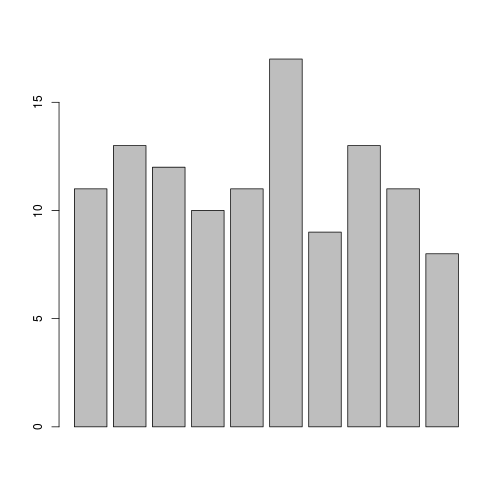### Note long names off edge:
barplot(x, names = mynames, las = 2)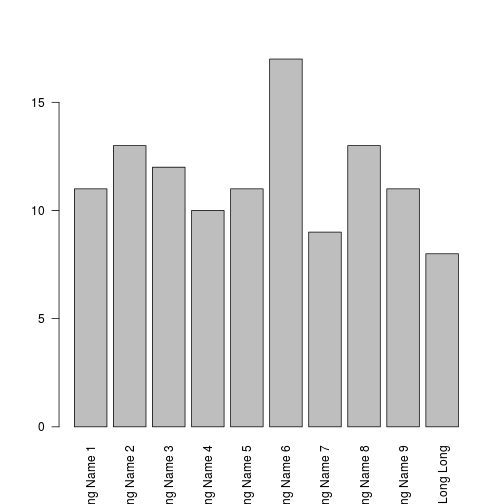### Abbreviate offers one solution
barplot(x, names = abbreviate(mynames), las = 2)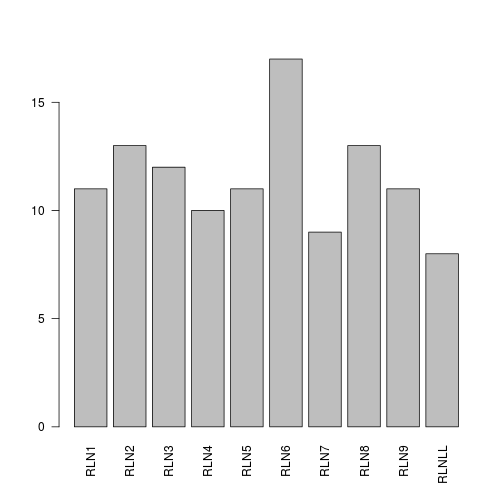### Other option is to reset margins
###default mar is c(5.1, 4.1, 4.1, 2.1)

### Lets make the bottom margin larger
par(mar = c(15, 4.1, 4.1, 2.1))
barplot(x, names = mynames, las = 2)

### Now the bottom is finished. But I'd like a legend.

legend("topleft", legend = c("A really long label", "B", "Cee What I can do?", "D"), col = 1:2)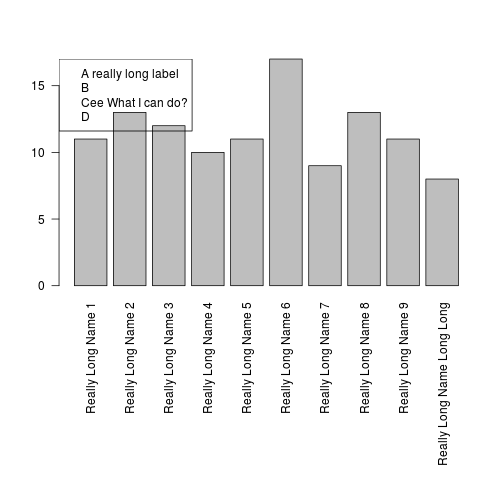### My bar hits the legend. How to fix?

### It is not sufficient to simply "move the legend" up
### because then it runs off the edge of the graph
barplot(x, names = mynames, las = 2)

legend(2, 22, legend = c("A really long label", "B",
"Cee What I can do?", "D"), col = 1:2)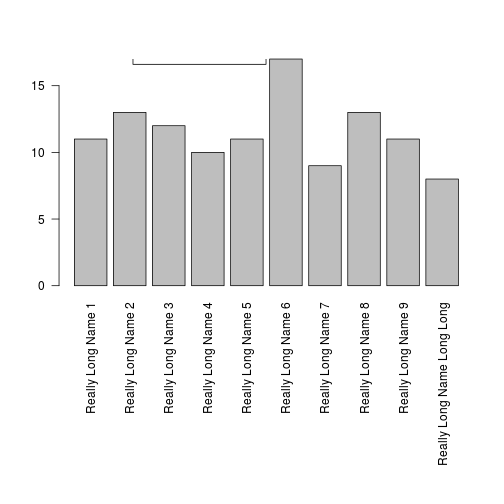### By itself, changing the top margin does not help.
### It is also vital to set the xpd parameter to T so that
### R will draw outside the main plot region
par(mar = c(10, 4.1, 8.1, 2.1))
par(xpd = T)
barplot(x, names = mynames, las = 2)

legend(2, 22, legend = c("A really long label", "B",
"Cee What I can do?", "D"), col = 1:2)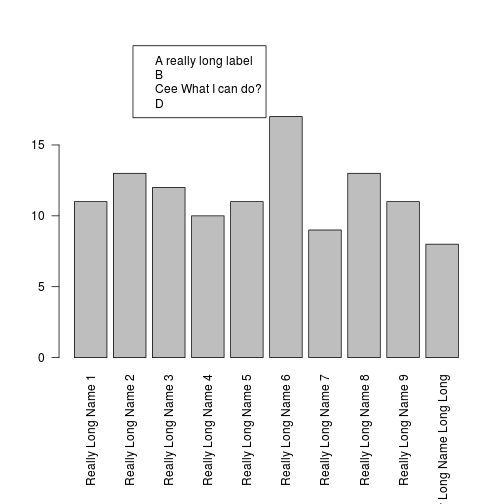### Now lets gain some control on the colors and bars
### The default colors are so dark.  You can't write on top
### of them.  You can grab shades of gray like "gray30" or such.

### I'll just specify 5 colors I know will work. I prefer
### the light gray colors because they can be written over.
mycols <- c("lightgray", "gray60", "gray80", "gray90", "gray70")
barplot(x, names = mynames, las = 2, col = mycols)
legend(2, 20, legend = c("A", "B", "C", "D", "E"), fill = mycols)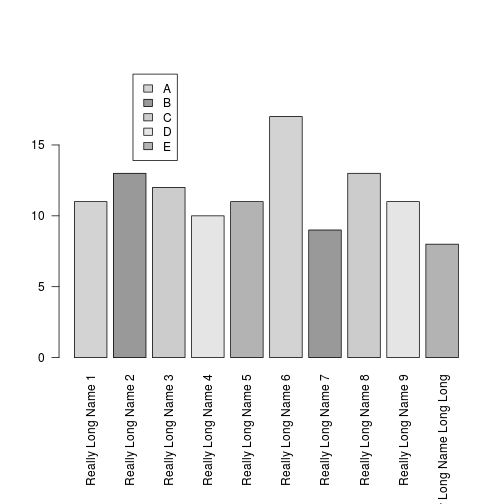## Note the 5 colors are "recycled" in the graph.

## It is up to you to decide if you want 10 separate colors for 10
## separate bars. I use the "rainbow" function to get 10 unique colors
## if I'm feeling lively:

rainbow(10)

##   "#FF0000FF" "#FF9900FF" "#CCFF00FF" "#33FF00FF" "#00FF66FF"
##   "#00FFFFFF" "#0066FFFF" "#3300FFFF" "#CC00FFFF" "#FF0099FF"

## But usually I'm gray, and you can ask for 10 shades of gray like this:
myGrays <- gray.colors(10)

## Note the output there is unmanageable because the legend is too long.
barplot(x, names = mynames, las = 2, col = myGrays)
legend(0.5, 20, legend = LETTERS[1:10], fill = mycols)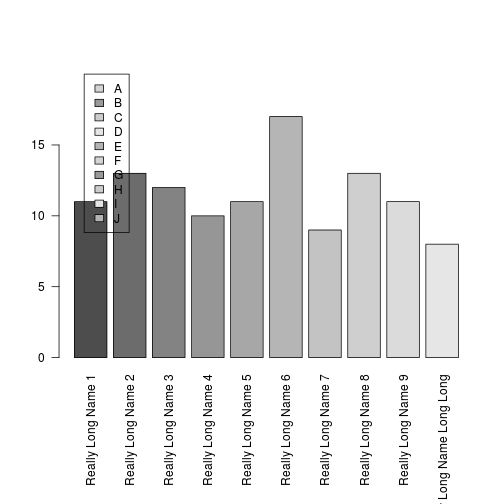## I'm not going down that path right now, I don't think color-based
## legends are so helpful, unless we are writing for Time Magazine.

### I don't like the solid shades so much.
### Let's fill up the bars with angled lines.
### density determines number of lines inside the bar.

myden <- c(60, 20, 30, 40, 25)
### angles determines the direction of lines inside bars
myangles <- c(20, -30, 60, -40, 10)
## These are "recycled" across 10 bars. Maybe you don't like that, so fix it :)

barplot(x, names = mynames, las = 2, col = mycols, density = myden, angle = myangles)
legend(1, 20, legend = c("A", "B", "C", "D", "E"), density = myden, angle = myangles)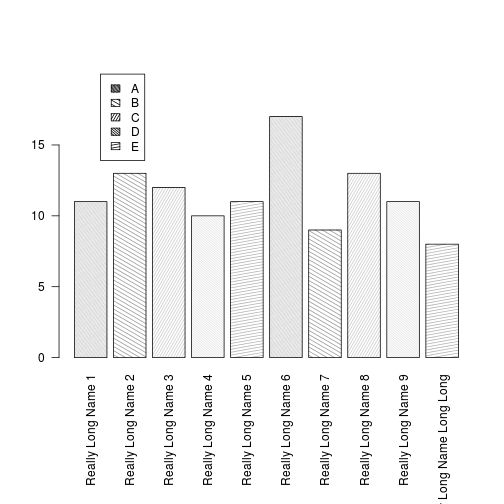### for my coupe de grace, lets do some writing in the bars.

### Recall from Verzani you can get the x coordinates from the barplot
barPositions <- barplot(x, names = mynames, las = 2, col = mycols, density = myden, angle = c(20, 60, -20))

barPositions

##       [,1]
##  [1,]  0.7
##  [2,]  1.9
##  [3,]  3.1
##  [4,]  4.3
##  [5,]  5.5
##  [6,]  6.7
##  [7,]  7.9
##  [8,]  9.1
##  [9,] 10.3
## [10,] 11.5

### The text option srt = 90 turns the text sideways

### I'm just guessing that bars 1 and 8 should be labeled
### at coordinates 5 and 5

text (barPositions, 5, "my first bar is great", srt = 90)

text (barPositions, 5, "but 8 is greater", srt = 90)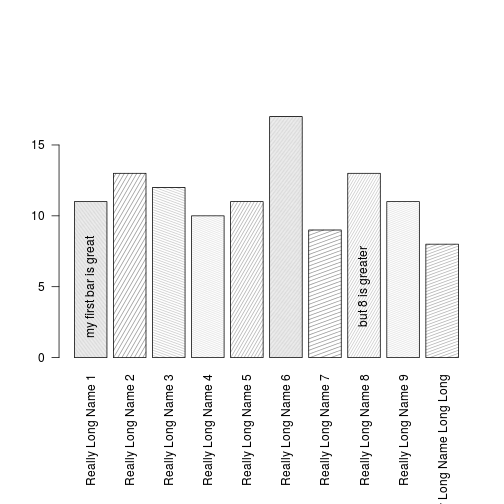### Recently I had the problem of drawing a "clustered" bar chart.
### Lets suppose our x variable really represents responses from
### 2 groups of respondents, Men and Women.

## Create the matrix
xmatr <- matrix(x, nrow = 5)

### Use beside = T to cause barplot to treat each column
### of values as a group
barplot(xmatr, beside = TRUE, names = c("Men", "Women"))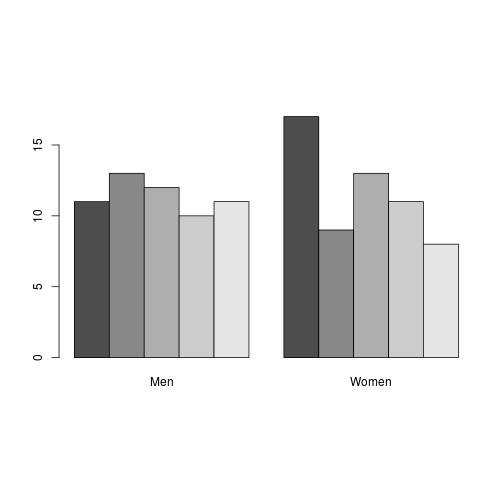valueNames <- c("Very Strong", "Somewhat Strong", "Not Strong", "Somewhat Weak", "Very Weak")

### Use mtext to write in the margin so that labels on plot are nice.

par(mar = c(10, 4.1, 5.1, 2.1))

bp <- barplot(xmatr, beside = TRUE, names = NULL)

### bp contains the positions of the bars.
mtext(valueNames, side = 1, las = 3, at = bp)

mtext(c("Men", "Women"), side = 1, at = c(bp, bp), line = 8, cex = 2)

### Adding numbers to the bars may clarify
text ( bp, as.vector(xmatr), labels = as.vector(xmatr), pos = 1)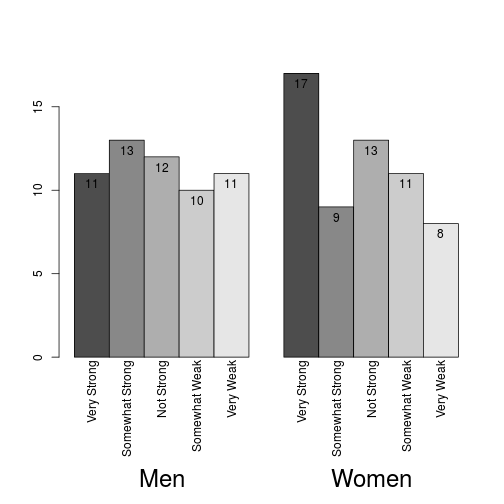### However, the default color is too dark.

### Retrieve the first 5 items from the default grey color scale

gc <- grey.colors(5)

### Replace the first one with a lighter gray

gc <- "gray80"

bp <- barplot(xmatr, beside = TRUE, names = NULL, col = gc)

### bp contains the positions of the bars.
mtext(valueNames, side = 1, las = 3, at = bp)

mtext(c("Men", "Women"), side = 1, at = c(bp, bp), line = 8, cex = 2)

### Adding numbers to the bars may clarify
text ( bp, as.vector(xmatr), labels = as.vector(xmatr), pos = 1)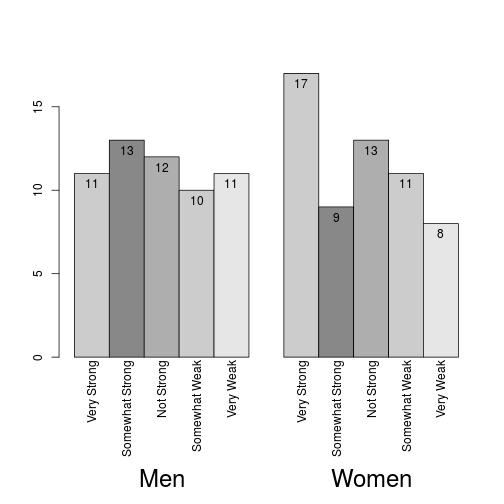### That's OK for me.  I wonder if I might not just write inside the
### bars. Hmmm.

bp <- barplot(xmatr, beside = TRUE, names = c("Men", "Women"), col = gc)

text(bp, 0.5*as.vector(xmatr), valueNames, srt = 90)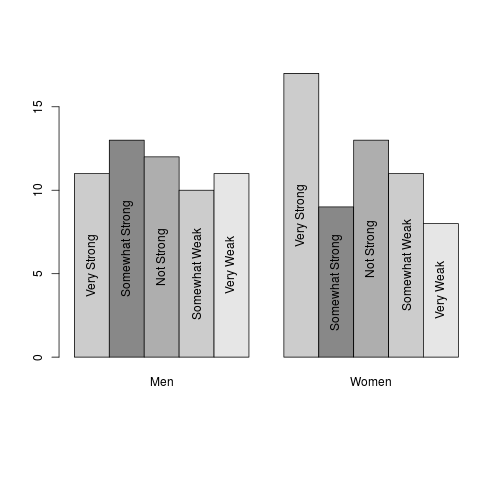### Hm. That's OK, but maybe I'd pref uniform vertical
### placement of text.

bp <- barplot(xmatr, beside = TRUE, names = c("Men", "Women"), col = gc)

text(bp, 2, valueNames, srt = 90, pos = 4)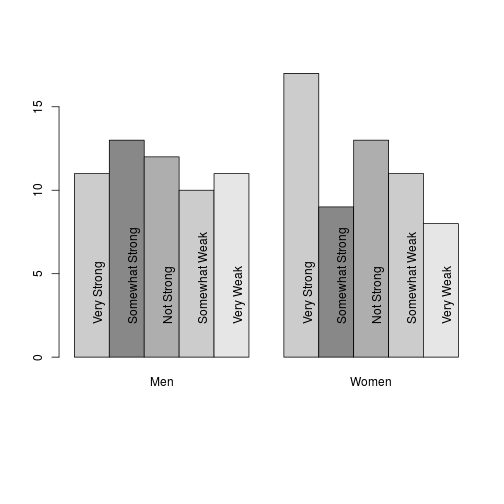## Hell, now the text is aligned vertically, but not centered
## in the bar. I can't figure out all of the details concerning
##

## I'm not able to say for sure what the best fix might be.
## I can either manipulate the x placement like so

bp <- barplot(xmatr, beside = TRUE, names = c("Men", "Women"), col = gc)
text(bp-0.25, 2, valueNames, srt = 90, pos = 4)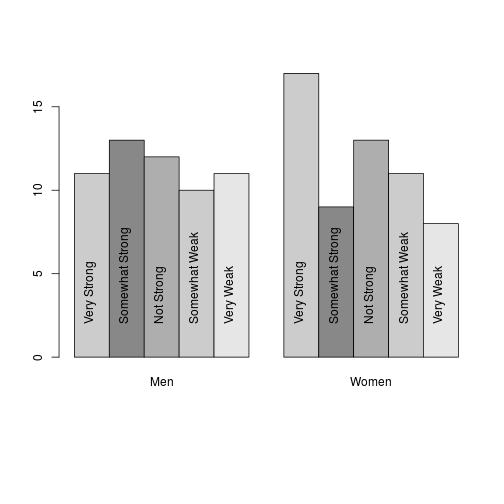## Or use the offset option in the text command.
## I do not understand why this particular value works.

bp <- barplot(xmatr, beside = TRUE, names = c("Men", "Women"), col = gc)
text(bp, 2, valueNames, srt = 90, pos = 4, offset = -0.15)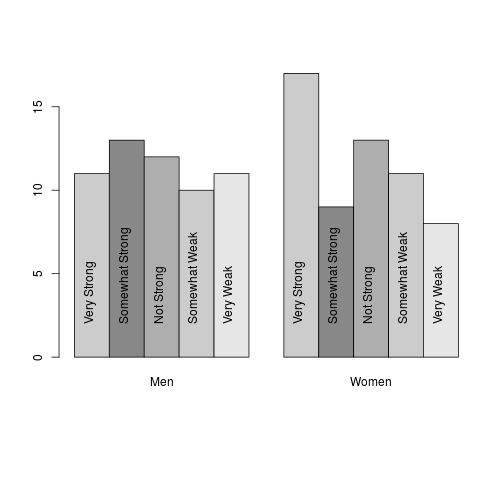## Don't forget: When save this into a file, the graph may be
## re-sized and text might "overflow" the boxes.  That's especially
## likely if you have a large graph on the screen and then try to
## save the file with dev.copy(pdf, ...)  That will resize some
## things, but not all.

## Here is the way to protect yourself if you are working
## interactively.  Create a fresh "screen" device that is the same
## size--in inches--as the output file. On any platform (after R 2.0,
## I think), this will pop up a new screen device of the desired size
##
## I'm leaving it commented out, because it interferes with some
## automated processing tools I use for these files, but you should
## try it.
##
## dev.new(width = 6, height = 6)

## After you do that, run the par command again. The par command has
## to be executed again each time a device is created. That applies to
## the most recently created device.

p <- barplot(xmatr, beside = TRUE, names = c("Men", "Women"), col = gc)
text(bp-0.25, 2, valueNames, srt = 90, pos = 4)## If that looks OK on the screen device, then do this to save it in a
## file.

pdf(file = "mybar-1.pdf", height = 6, width = 6, onefile = FALSE,
paper = "special", family = "Times")
p <- barplot(xmatr, beside = TRUE, names = c("Men", "Women"), col = gc)
text(bp-0.25, 2, valueNames, srt = 90, pos = 4)
dev.off()

## pdf
##   2

## note that the par settings have shifted back to the defaults.
## Each device is separate.
## As a result, the barplot we created before with large margins would
## make a horrible plot if you forgot to insert the par() commands here:

pdf(file = "mybar-2.pdf", height = 6, width = 6, onefile = FALSE,
paper = "special", family = "Times")
par(mar = c(10, 4.1, 5.1, 2.1))
bp <- barplot(xmatr, beside = TRUE, names = NULL, col = gc)

## bp contains the positions of the bars.
mtext(valueNames, side = 1, las = 3, at = bp)
mtext(c("Men", "Women"), side = 1, at = c(bp, bp), line = 8, cex = 2)

## Adding numbers to the bars may clarify
text ( bp, as.vector(xmatr), labels = as.vector(xmatr), pos = 1)
dev.off()

## pdf
##   2

## Finally, suppose you make a mistake of specifying your
## output device size too small. The graph I made before
## with the text in the bars looked good on the screen,
## but it looks bad in a smaller output device. The text
## does not match the bars in this example.

pdf(file = "mybar-4.pdf", height = 4, width = 4, onefile = F,
horizontal = F, paper = "special", family = "Times")

## Error: unused argument (horizontal = F)

bp <- barplot(xmatr, beside = TRUE, names = c("Men", "Women"), col = gc)
text(bp-0.25, 2, valueNames, srt = 90, pos = 4)dev.off()

## null device
##           1